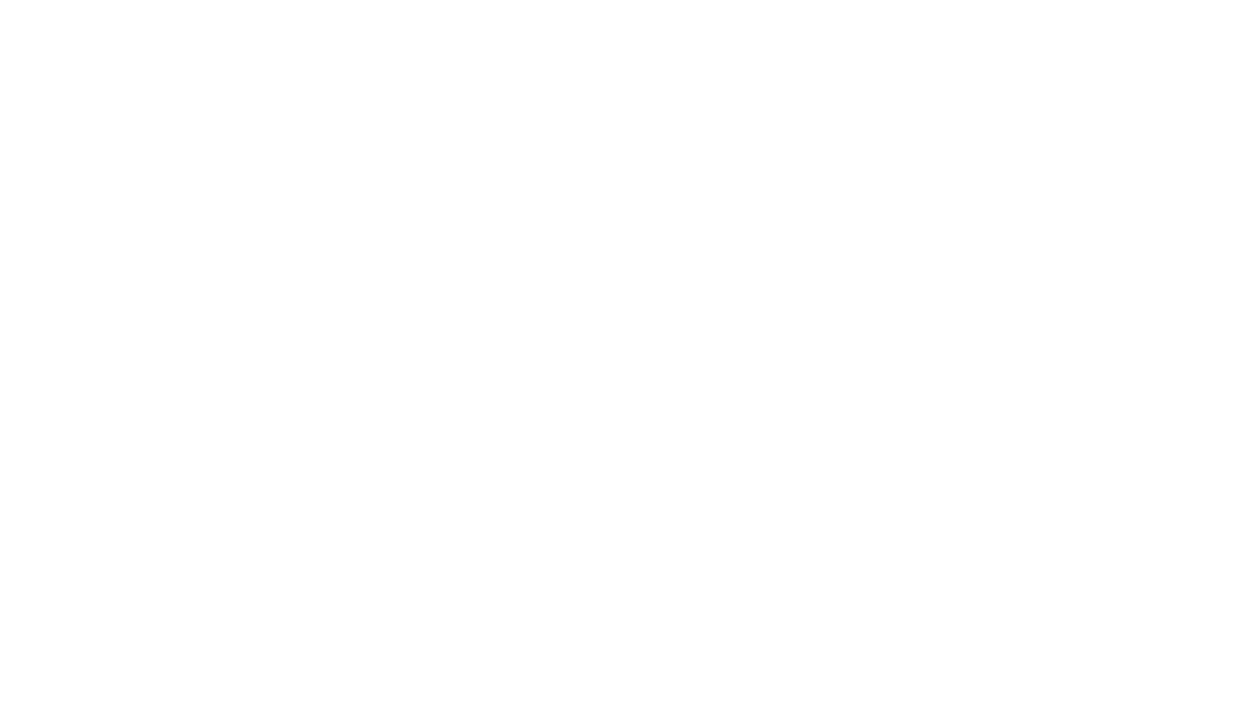# Loop programming exercises and solutions in C++

Let us see a list of important Loop programming exercises and solutions in C++.

## For loop

For loop is a methodology to use a piece of code again and again until the given condition remains true. Loop will terminate when the given condition will false.

For loop have three parts. Loop initialization, Condition, and increment or decrement of a loop.

1. Program to find the length of a number in C++ (CPP). Solution
2. Program to find the square root of a number in C++. Solution
3. Factorial Program in C, C++ (C Plus Plus, CPP) with flow chart. Solution
4. Fibonacci Series Program in C++ and C with flowchart. Solution
5. Palindrome Program in C, C++, C Plus Plus (CPP) with flow chart. Solution
6. Program to the Print sum of the number of Series in C Plus Plus (CPP, C++) and C with Flowchart. Solution
7. C++ and C Program to Find the Number of Vowels, Digits, Consonants and White Spaces in a String with flowchart. Solution
8. Program in C++, C to display the reverse of a number with a flowchart. Solution
9. Write a program that lets the user enter the total rainfall for each of 12 months
10. Write a C++ program to print a triangle of prime numbers up to given number of lines of the triangle
11. Write a C++ program to print all numbers between a and b (a and b inclusive) using for loops
12. Write a simple C++ program to find the mean value

## while loop

The while loop is a methodology to use a piece of code again and again until the given condition remains true. Loop will terminate when the given condition will false.

Just lie for loop, the while loop has three parts. 1. Loop initialization, 2. Condition, and 3. increment or decrement of a loop.

1. Program of the sum of all digits of a number in C, C Plus Plus (CPP, C++) with flow chart. the solution
2. converts a number into binary in C++and C with a flowchart. Solution
3. Program in C Plus Plus and C with an explanation to find the Greatest Common Division (GCD). Solution with flowchart. Solution
4. C++ Program to convert Octal to decimal number using while loop. Solution
5. Write a program using integers usernum and x as input, and output usernum divided by x four times.
6. Write a C++ program to add two numbers without using the addition operator
7. Write a C++ program that simulates the rolling of two dice

## Do while loop

In Do, while loop, first one time we execute the do part of the loop, and then we check the condition. It means that if the condition is false then still do part execute one time.

1. C++ Program to Display English Alphabets from  A-Z. Solution
2. Write a program in C++ to display the multiplication table vertically from 1 to n. Solution
3. C++ Program to Find the Sum of Digits of a Number – C++ Program. Solution
4. Program in C++ to display the n terms of even natural number and their sum. Solution
5. C++ program to find the sum of all integer between 100 and 200 which are divisible by 9. Solution
6. Write a program in C++ to print Floyd’s Triangle. Solution
7. C++ program to print pyramid pattern of numbers.  Solution
8. C++ Program to display the n terms of odd natural number and their sum. Solution
9. Convert a binary number to octal in C++. Solution
10. c++ program to check whether a given number is a perfect number or not. Solution
11. C++ program to find HCF (Highest Common Factor) of two numbers. Solution
12. Program in C++ to convert a decimal number into binary without using an array. Solution
13. Program C++ to convert an octal number into binary. Solution
14. Write a c program to find out the sum of an A.P. series. Solution
15. Program in C++, C to display the reverse of a number with flowchart. Solution
16. Write the Octal to Decimal number program in C++. Solution
17. C++ Program right angle triangle with a number that will repeat a number in a row. Solution
18. C++ program to print rhombus or parallelogram star pattern. Solution
19. Program in C++ to make such a pattern like a pyramid with a number that will repeat the number in the same row. Solution
20. Write a program in C++ to Check Whether a Number can be Express as a Sum of Two Prime Numbers. Solution
21. C++ program to print mirrored right triangle star pattern. Solution
22. Decimal to octal number conversion program in C++. Solution
23. C++ program to print a hollow square or rectangle star pattern. Solution
24. C++ Program Sum of odd Natural Number. Solution
25. The sum of the first 10 natural numbers program in C++. Solution

## Prefix and postfix

1. Prefix and postfix increment and decrement in C++. Solution

## Strings

Remove Whitespaces from the program

## Arrays

Two’s complement of a binary number in C++

## Pointers

Write a program in C++ to add two numbers using pointers

## General Programs

Write a C++ program to Set Get Clear a particular bit in a given number## C Language Programs

Write a c program to reverse a given word using a function

write a C program to sort a 1d array using pointer by applying the bubble sort technique